# You Didn’t Know It, but You Really Want to Learn Sequent Calculus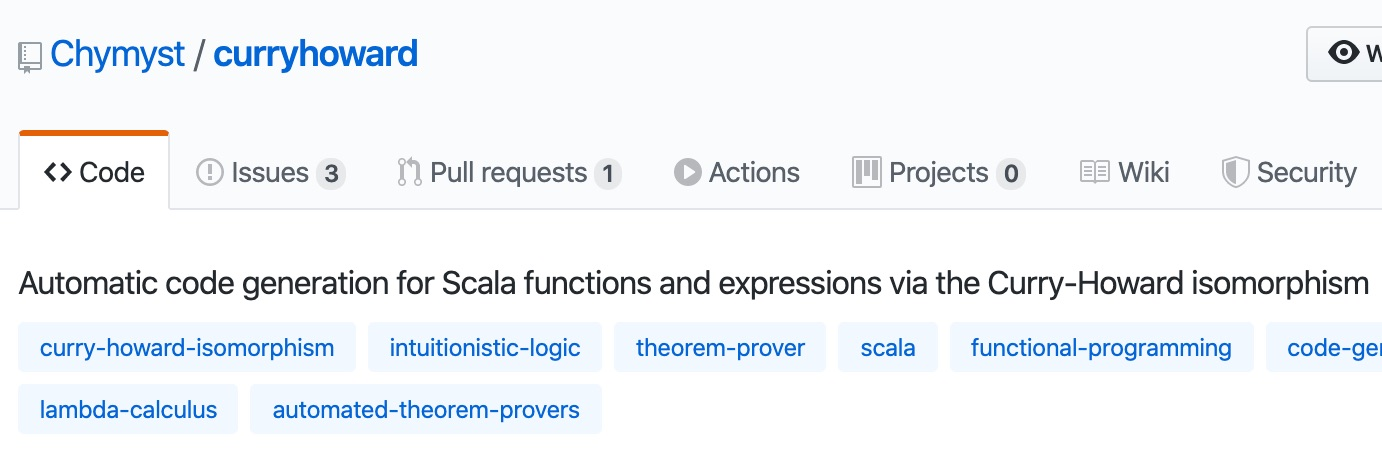ʘ‿ʘ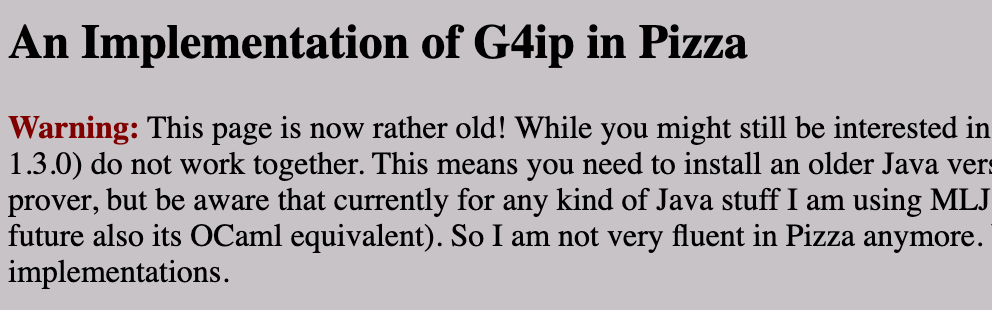(｡◕‿◕｡)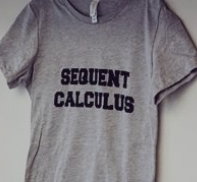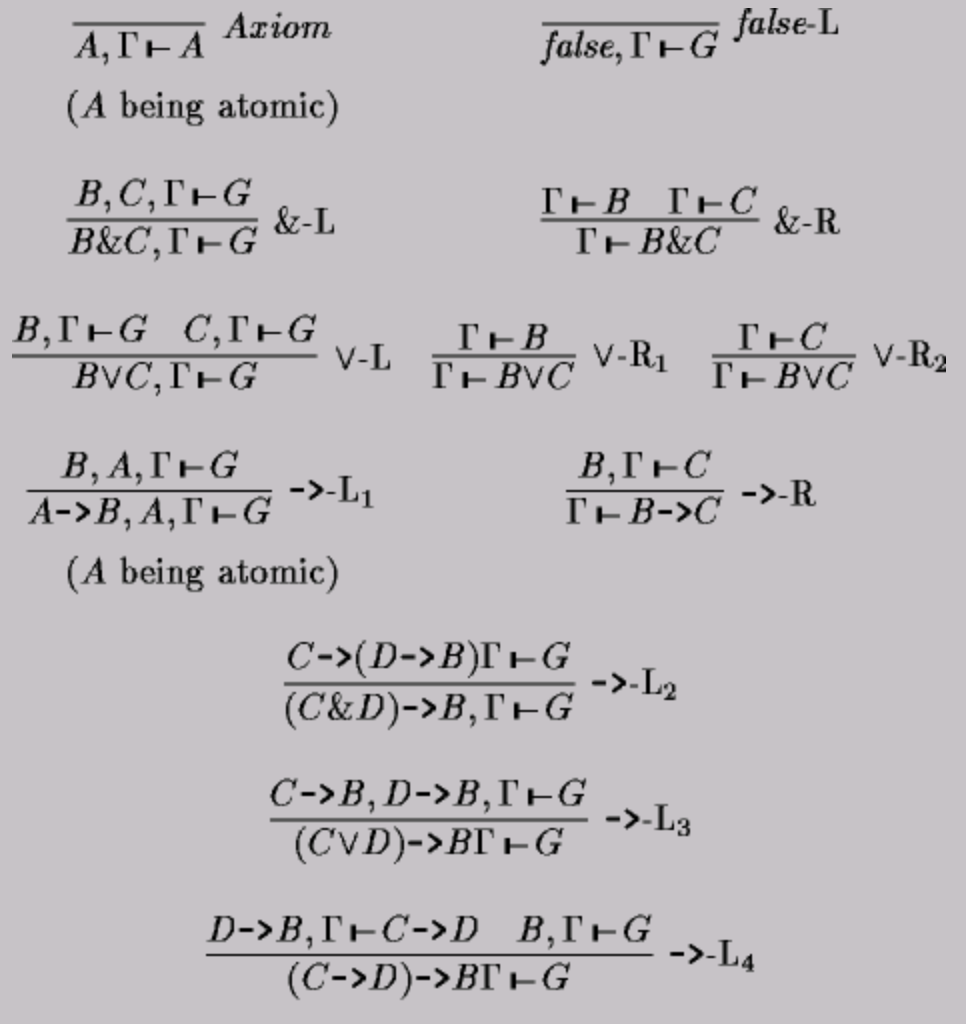Sequents? Proofs? WTH is LJT? Why did I start this!? ( ͡ಠ ʖ̯ ͡ಠ)
 Fake it 'til you make it! (but use programming strategies!) (ᵔᴥᵔ)

## Our Goal

given a type signature,
automatically derive a program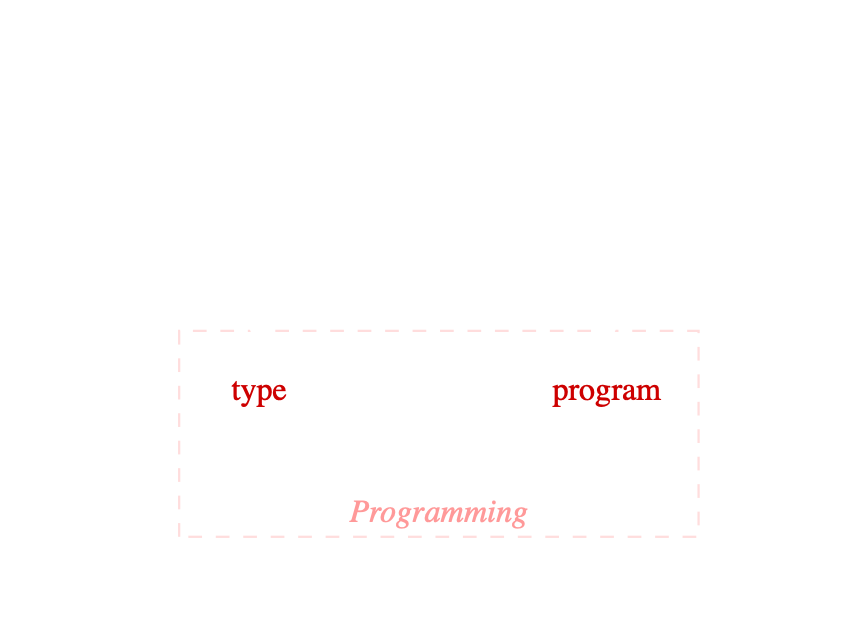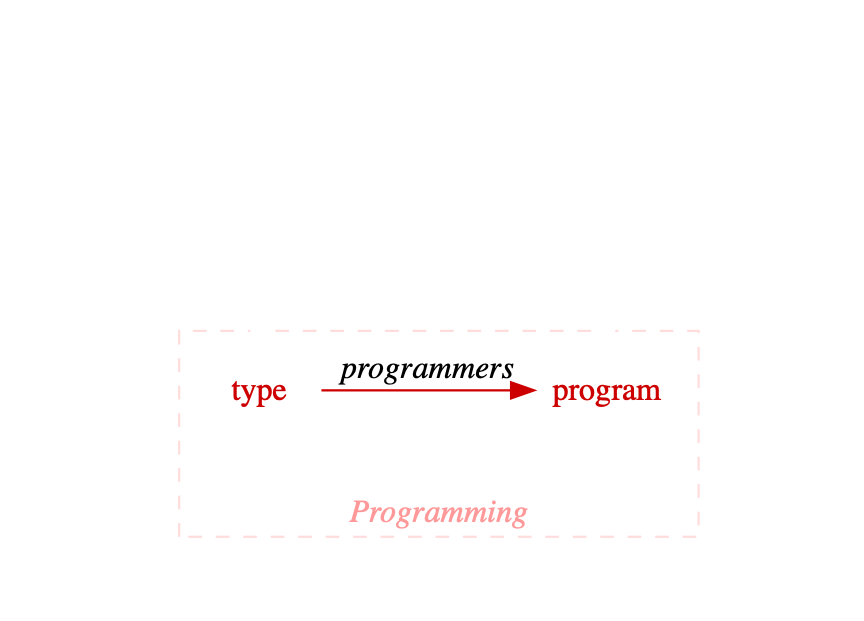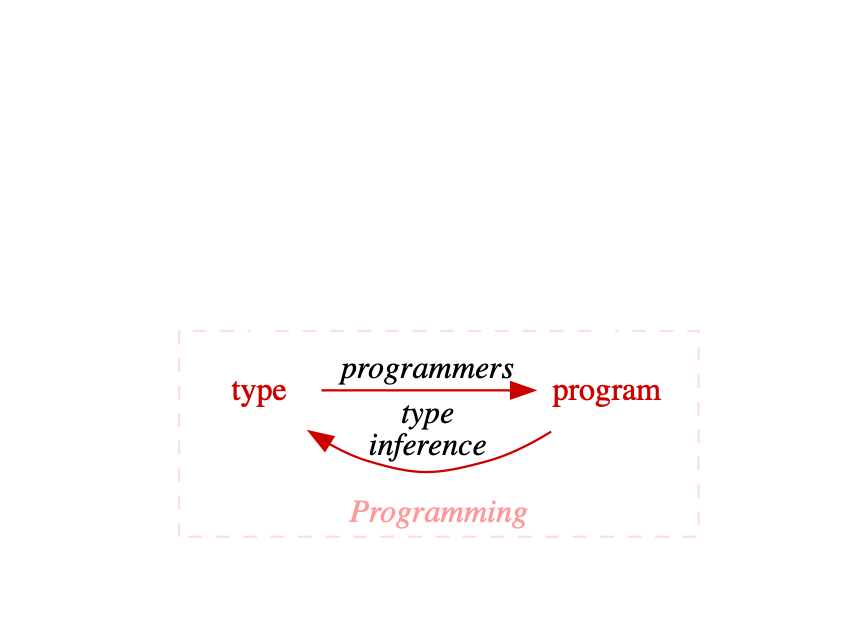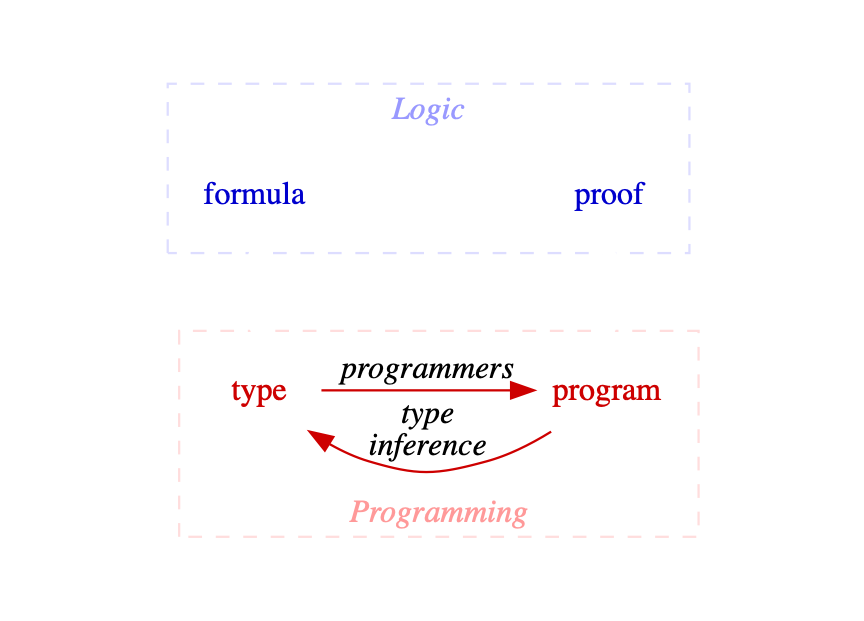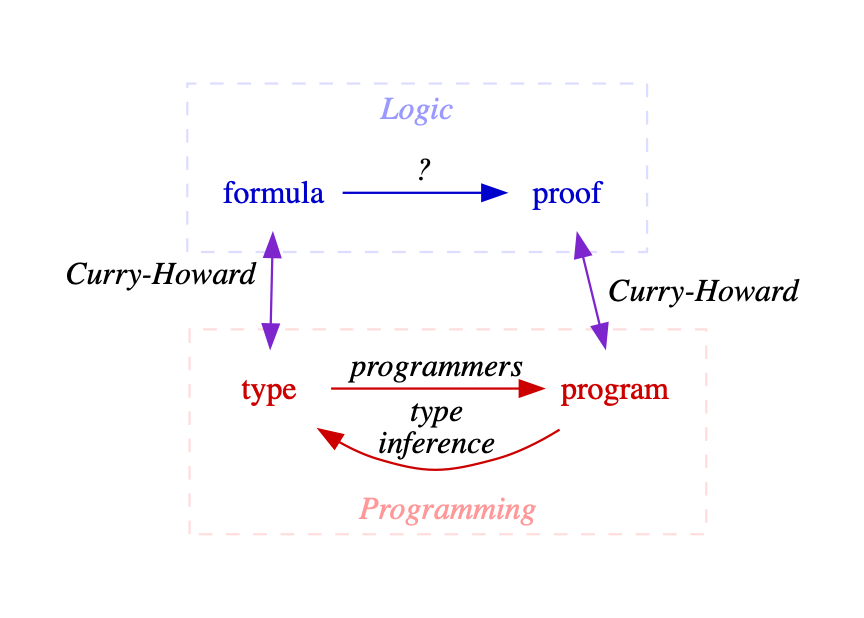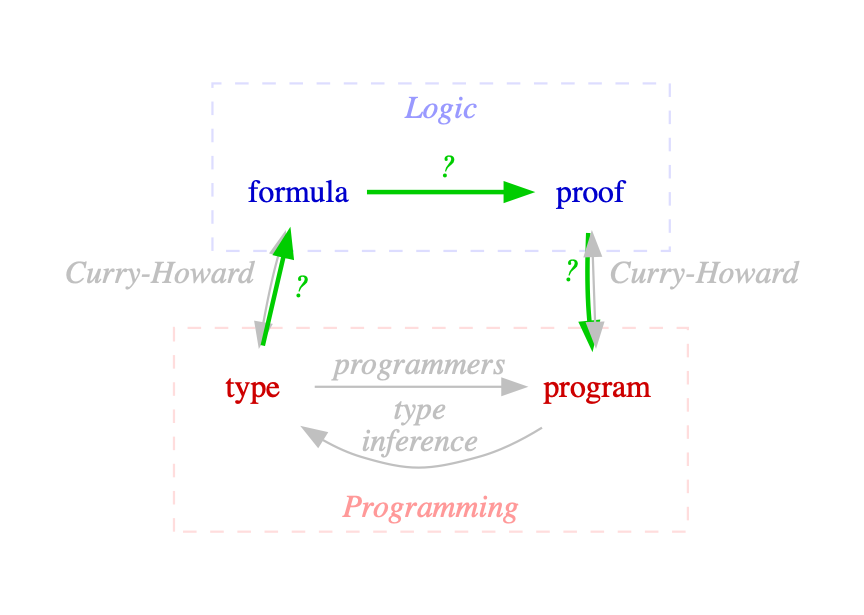## Types ⇒ Formulas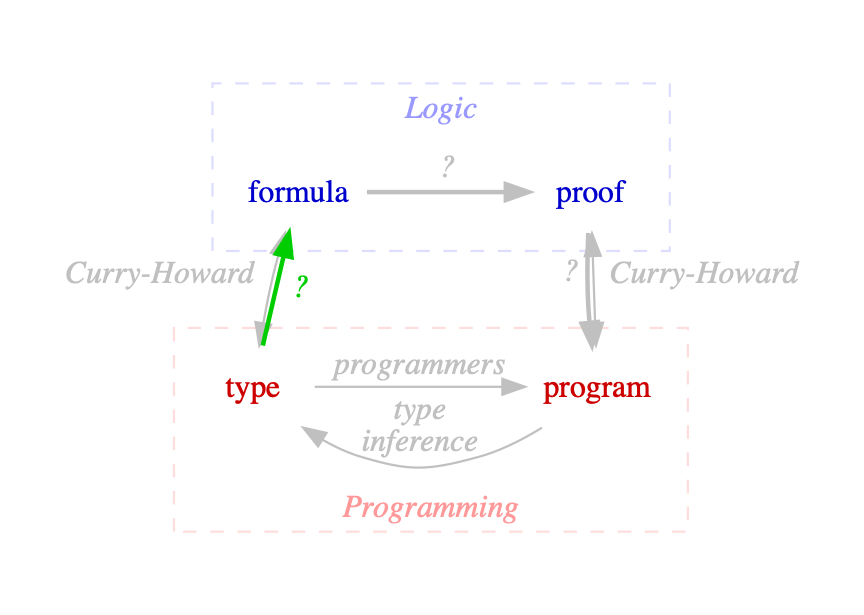TypeFormula

`Unit`

🔊 "true"

`Nothing`

🔊 "false"

`A`, …​

`A`, …​

`(A, B)`

`A ∧ B` 🔊 "and"

`Either[A, B]`

`A ∨ B` 🔊 "or"

`A ⇒ B`

`A ⇒ B` 🔊 "implies"

TODO: type params, universals, existentials.

``````sealed trait Formula

object Formula {
sealed trait Atomic extends Formula (1)
case object True extends Atomic
case object False extends Atomic
case class Type(name: String) extends Atomic
case class And(fst: Formula, snd: Formula) extends Formula
case class Or(inl: Formula, inr: Formula) extends Formula
case class Implies(from: Formula, to: Formula) extends Formula
}``````

Reification Algebraic Data Type

## Example: Type ⇒ Formula

``````type Example = (A, Either[B, C])

val example =
Formula.And(
Formula.Type("A")
Formula.Or(
Formula.Type("B"),
Formula.Type("C")))``````

## 💯Pretty-Printing with `Show`

``````  implicit val show: Show[Formula] = {
case True              => "⊤"
case False             => "⊥"
case Type(name)        => name
case And(fst, snd)     => show"($fst ∧$snd)"
case Or(inl, inr)      => show"($inl ∨$inr)"
case Implies(from, to) => show"($from ⇒$to)"
}``````

## 💯Pretty-Printing with `Show`

``````// (A, Either[B, C])
val example =
Formula.And(
Formula.Type("A")
Formula.Or(
Formula.Type("B"),
Formula.Type("C")))
example.show``````
`(A ∧ (B ∨ C))`

## Type ⇒ Formula

``````trait ToFormula[A] {
def toFormula(): Formula
}``````

Typeclass

## Type ⇒ Formula

``````trait A
trait B
trait C

implicit val toFormulaA = ToFormula.reify[A]
implicit val toFormulaB = ToFormula.reify[B]
implicit val toFormulaC = ToFormula.reify[C]

ToFormula[(A, Either[B, C])].toFormula.show``````
`(A ∧ (B ∨ C))`

## Type ⇒ Formula

``````  implicit def product2[A, B](
implicit toA: ToFormula[A],
toB: ToFormula[B]
): ToFormula[(A, B)] =
() => Formula.And(toA.toFormula(), toB.toFormula())

implicit def sum2[A, B](
implicit toA: ToFormula[A],
toB: ToFormula[B]
): ToFormula[Either[A, B]] =
() => Formula.Or(toA.toFormula(), toB.toFormula())``````

Typeclass Derivation

## Formulas ⇒ Proofs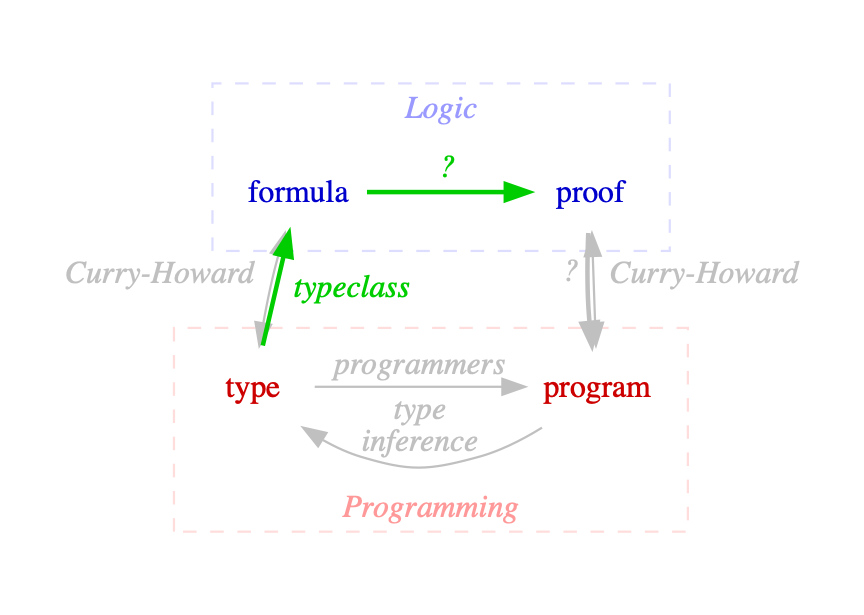``````trait Prover[Rule] {
def prove(formula: Formula): Proof[Rule]
}

sealed trait Proof[Rule] // TODO``````

`Unit`

🔊 "Give me a `Unit`."

`val proof = ()`

`A`

🔊 "Give me an `A`."

`val proof = ???`

`A ⇒ A`

🔊 "If you give me an `A`, I’ll give you an `A`."

`val proof = (a: A) ⇒ a`

`(A, B) ⇒ A`

🔊 "If you give me an `A` and a `B`,
I’ll give you an `A`."

`val proof = (a: A, _: B) ⇒ a`

`A ⇒ Either[A, B]`

🔊 "If you give me an `A`, I’ll give you an `A` or `B`."

`val proof = (a: A) ⇒ Left(a)`

How did you know how to do that?

Can we teach a computer?

## More Complex Formulas

`(A, B) ⇒ Either[A, C]`

no

 ↙︎ ↓ extract args `(A, B)` `Either[A, C]` no
 un-pair ↓ `A, B` `Either[A, C]` no
 ↓ choose `A` `A, B` `A` Yes!
If we had ⊢ Could we produce?

`⊢ (A, B) ⇒ Either[A, C]`

no

 ↓ extract args `(A, B) ⊢ Either[A, C]` no
 ↓ un-pair `A, B ⊢ Either[A, C]` no
 ↓ choose `A` `A, B ⊢ A` Yes!

## Sequents

 Gamma |-- Delta 🔊 "If the premise Gamma is true, then the conclusion Delta is true."
 |-- 🔊 "entails"
``````case class Sequent(
premises: List[Formula],
conclusion: Formula)``````

## Deduction ↑

(A |-- B)/(|-- A => B)

🔊 "if we conclude |-- A => B,
then we can presume A |-- B".

You can also go the other way: Reduction ↓

## Inference Rules

 (Gamma, A |-- B)/(Gamma |-- A ⇒ B)R⇒ 🔊 "if we conclude |-- A ⇒ B, then we can presume A |-- B". Gamma 🔊"whatever" R=> 🔊 "the rule R⇒"
``````case LK.`R⇒` => {
case Sequent(g, Formula.Implies(a, b)) =>
Deduction.Success(
rule,
NonEmptyList.of(Sequent(a :: g, b)))
}``````
 A collection of rules is known as a system. ``````/** A [[System]] contains a set of rules. */ case class System[Rule](rules: NonEmptySet[Rule])``````
 Gerhard Gentzen created the LK (klassische Prädikatenlogik) system in 1934. ``````sealed trait LK object LK { /** * -------- Id * Γ, A ⊢ A */ case object Id extends LK ...``````
 Each rule specifies a possible deduction. ``````case Id => { case Sequent(ps, c) if ps contains c => Deduction.Discharged(rule) }``````
 A deduction can be stuck, discharged (done), or success (produces one or more new, but simpler, sequents). ``````sealed trait Deduction[Rule] case class Stuck[Rule](rule: Rule) extends Deduction[Rule] case class Discharged[Rule](rule: Rule) extends Deduction[Rule] case class Success[Rule](rule: Rule, sequents: NonEmptyList[Sequent]) extends Deduction[Rule]``````
 A prover attempts to prove a sequent. ``````trait Prover[Rule] { def prove(sequent: Sequent): Proof[Rule] }``````
``````val sequent =
Sequent.conclude[Tuple2[A, B] => Either[A, C]]

Prover[LK].prove(sequent).prune.toTree.show``````
```( ⊢ ((A ∧ B) ⇒ (A ∨ C))
(R⇒
((A ∧ B) ⊢ (A ∨ C)
(L∧
(A, B ⊢ (A ∨ C)
(R∨1
(A, B ⊢ A
(Id))))))))```
 $\dfrac{\dfrac{\dfrac{\dfrac{}{A, B \vdash A}Id}{A, B \vdash A \vee C}R\vee1}{A \wedge B \vdash A \vee C}L\wedge}{\vdash A \wedge B \Rightarrow A \vee C}R\Rightarrow$ ```( ⊢ ((A ∧ B) ⇒ (A ∨ C)) (R⇒ ((A ∧ B) ⊢ (A ∨ C) (L∧ (A, B ⊢ (A ∨ C) (R∨1 (A, B ⊢ A (Id))))))))```

Remember everybody, only in computer science do trees grow upside-down!

## Proofs ⇒ Programs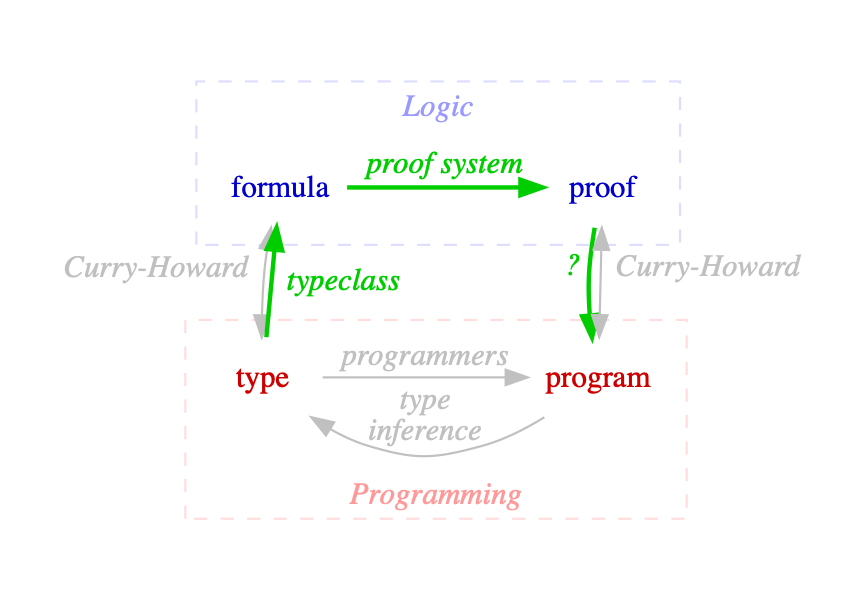## Basic Idea: Fold Proofs into Programs

`(A, B) ⇒ Either[A, C]`

`(a, _) ⇒ Left(a)`

↙︎ ↓ extract args

`(A, B)`

`Either[A, C]`

un-pair ↓

`A, B`

`Either[A, C]`

`(a, _) ⇒ Left(a)`

↓ choose `A`

`_ andThen Left(_)`

`A, B`

`A`

`(a, _) ⇒ a`

## (∩｀-´)⊃━☆ﾟ.*･｡ﾟ

`// TODO: "Simply Typed Lambda Calculus" ADT and intepreter`
`// TODO: macro to build programs at compile-time.`

## Summary

 While not originally popular with t-shirt collectors in 1934, sequent calculus is definitely cool.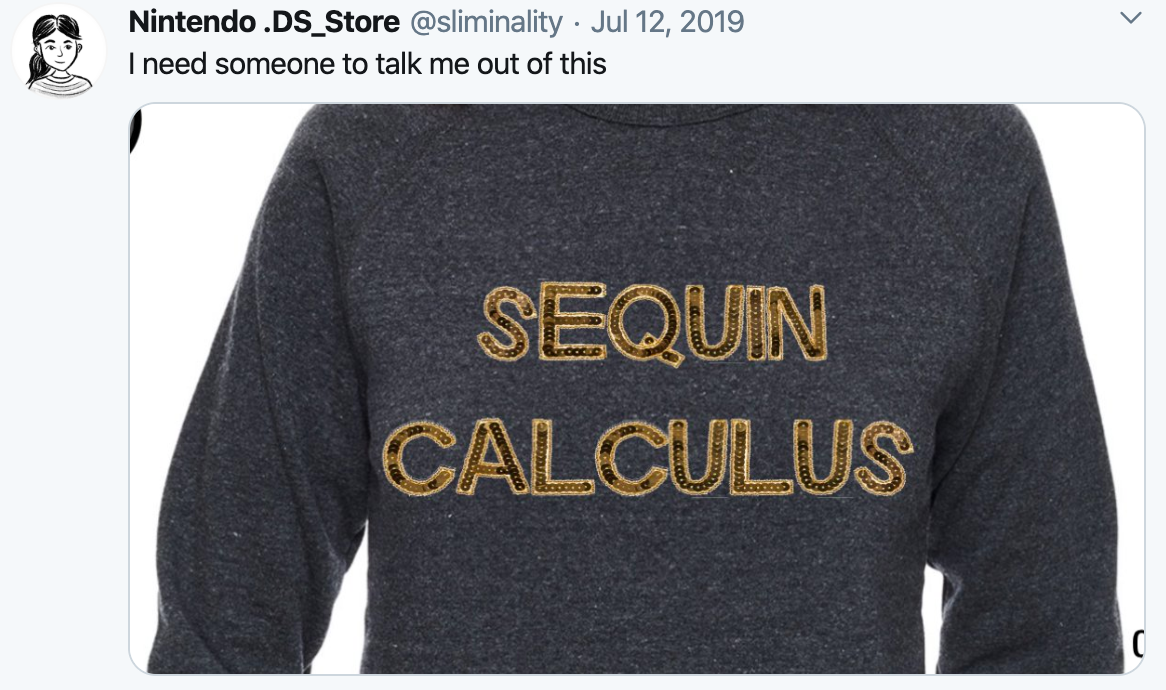Curry-Howard lets us translate our programming problems into logic problems, and back. And logic problems are solved with sequent calculus.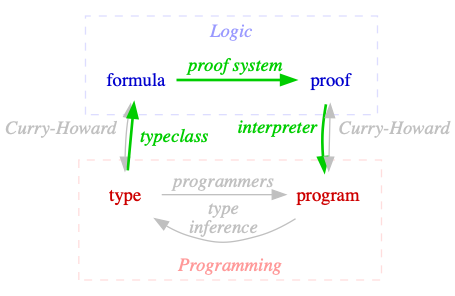## type ⇒ program in 3 Steps

 type ⇒ formula reification algebraic data type typeclass typeclass derivation formula ⇒ proof reification algebraic data type structural recursion typeclass interpreter proof ⇒ program interpreter structural recursion

## Thank you!

Hire us to teach your team! ☝︎